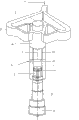# CN100523834C - 用于八毫米波段电介质测量的圆波导驻波测量装置 - Google Patents

## Info

Publication number
CN100523834C
CN100523834C CNB2007100677394A CN200710067739A CN100523834C CN 100523834 C CN100523834 C CN 100523834C CN B2007100677394 A CNB2007100677394 A CN B2007100677394A CN 200710067739 A CN200710067739 A CN 200710067739A CN 100523834 C CN100523834 C CN 100523834C
Authority
CN
China
Prior art keywords
circular waveguide
waveguide
standing wave
mode
piston
Prior art date
Legal status (The legal status is an assumption and is not a legal conclusion. Google has not performed a legal analysis and makes no representation as to the accuracy of the status listed.)
Expired - Fee Related
Application number
CNB2007100677394A
Other languages
English (en)
Other versions
CN101105512A (zh
Inventor

Current Assignee (The listed assignees may be inaccurate. Google has not performed a legal analysis and makes no representation or warranty as to the accuracy of the list.)
Zhejiang University ZJU
Original Assignee
Zhejiang University ZJU
Priority date (The priority date is an assumption and is not a legal conclusion. Google has not performed a legal analysis and makes no representation as to the accuracy of the date listed.)
Filing date
Publication date
Application filed by Zhejiang University ZJU filed Critical Zhejiang University ZJU
Priority to CNB2007100677394A priority Critical patent/CN100523834C/zh
Publication of CN101105512A publication Critical patent/CN101105512A/zh
Application granted granted Critical
Publication of CN100523834C publication Critical patent/CN100523834C/zh
Expired - Fee Related legal-status Critical Current
Anticipated expiration legal-status Critical

## Images

•## Description

$\frac{j\mathrm{tan}\frac{2\mathrm{πl}}{{\mathrm{λ}}_{g}}+\frac{{Z}_{2}}{{Z}_{1}}\mathrm{tanh}{\mathrm{γ}}_{2}d}{1+j\frac{{Z}_{1}}{{Z}_{2}}\mathrm{tan}\frac{2\mathrm{πl}}{{\mathrm{λ}}_{g}}\mathrm{tanh}{\mathrm{γ}}_{2}d}=\frac{\frac{{E}_{\mathrm{min}}}{{E}_{\mathrm{max}}}-j\mathrm{tan}\frac{2\mathrm{π}{x}_{0}}{{\mathrm{λ}}_{g}}}{1-j\frac{{E}_{\mathrm{min}}}{{E}_{\mathrm{max}}}\mathrm{tan}\frac{2\mathrm{π}{x}_{0}}{{\mathrm{λ}}_{g}}};---\left(1\right)$

Z2/Z1＝μrγ12＝μrj2π/(γ2λg)，                    (2)
${\mathrm{γ}}_{1}=j\sqrt{{\left(\frac{\mathrm{ω}}{c}\right)}^{2}-{\left(\frac{3.832}{a}\right)}^{2}}=j\frac{2\mathrm{π}}{{\mathrm{λ}}_{g}},---\left(3\right)$
${\mathrm{γ}}_{2}=j\sqrt{{\left(\frac{\mathrm{ω}}{c}\right)}^{2}\left(\left({\mathrm{ϵ}}_{r}{\mathrm{μ}}_{r}\right)′-j\left({\mathrm{ϵ}}_{r}{\mathrm{μ}}_{r}\right)′′\right)-{\left(\frac{3.832}{a}\right)}^{2}},---\left(4\right)$
${\mathrm{μ}}_{r}={\mathrm{μ}}_{r}^{′}-j{\text{μ}}_{r}^{′′},---\left(5\right)$
${\mathrm{ϵ}}_{r}={\mathrm{ϵ}}_{r}^{′}-j{\mathrm{ϵ}}_{r}^{′′}={\mathrm{ϵ}}_{r}^{′}\left(1-j\mathrm{tan}\mathrm{δ}\right)---\left(6\right)$
εrμr＝(εrμr)′-j(εrμr)＂。                   (7)

${\left(\frac{{Z}_{1}}{{Z}_{2}}\right)}^{2}=\frac{{\left(\frac{Z\left(0\right)}{{Z}_{1}}\right)}_{l}-j\mathrm{tan}\frac{2\mathrm{πl}}{{\mathrm{λ}}_{g}}-{\left(\frac{Z\left(0\right)}{{Z}_{1}}\right)}_{s}}{j\mathrm{tan}\frac{2\mathrm{πl}}{{\mathrm{λ}}_{g}}{\left(\frac{Z\left(0\right)}{{Z}_{1}}\right)}_{l}{\left(\frac{Z\left(0\right)}{{Z}_{1}}\right)}_{s}},---\left(8\right)$
$\mathrm{tanh}{\mathrm{γ}}_{2}d=\frac{{\left(\frac{Z\left(0\right)}{{Z}_{1}}\right)}_{l}-{\left(\frac{Z\left(0\right)}{{Z}_{1}}\right)}_{s}-j\mathrm{tan}\frac{2\mathrm{πl}}{{\mathrm{λ}}_{g}}}{-j\mathrm{tan}\frac{2\mathrm{πl}}{{\mathrm{λ}}_{g}}{\left(\frac{Z\left(0\right)}{{Z}_{1}}\right)}_{l}\frac{{Z}_{1}}{{Z}_{2}}};---\left(9\right)$

1)在活塞上放入厚度为d的介质样品，利用粗调机构将其推进到圆波导测量线的工作区、并准确定位在某一刻度M处，用细调机构调节活塞，找到纳瓦功率计的极小值读数Pmin和相应的细调测微器读数LS，接着再找到相邻点的纳瓦功率计极大值读数Pmax
2)取出介质样品，将粗调活塞重新准确地定位在M处，调节细调活塞，找到纳瓦功率计的两个相邻极小值读数处的细调测微器读数L01和L02
3)当介质样品直接与活塞接触(短路终端)时，从式(1)在l＝0下得到计算非磁性介质的相对复介电常数的公式：
$\frac{\mathrm{tanh}{\mathrm{γ}}_{2}d}{{\mathrm{γ}}_{2}d}=-j\frac{{\mathrm{λ}}_{g}}{2\mathrm{πd}}\frac{\frac{{E}_{\mathrm{min}}}{{E}_{\mathrm{max}}}-j\mathrm{tan}\frac{2\mathrm{π}{x}_{0}}{{\mathrm{λ}}_{g}}}{1-j\frac{{E}_{\mathrm{min}}}{{E}_{\mathrm{max}}}\mathrm{tan}\frac{2\mathrm{π}{x}_{0}}{{\mathrm{λ}}_{g}}},---\left(10\right)$
${\mathrm{γ}}_{2}=j\sqrt{{\left(\frac{\mathrm{ω}}{c}\right)}^{2}\left({\mathrm{ϵ}}_{r}^{′}-j{\mathrm{ϵ}}_{r}^{′′}\right)-{\left(\frac{3.832}{a}\right)}^{2}};---\left(11\right)$

4)当介质样品与活塞之间有λg/4间隙(开路终端)时，对于非磁性介质，式(1)简化为
$\frac{\mathrm{coth}{\mathrm{γ}}_{2}d}{{\mathrm{γ}}_{2}d}=-j\frac{{\mathrm{λ}}_{g}}{2\mathrm{πd}}\frac{\frac{{E}_{\mathrm{min}}}{{E}_{\mathrm{max}}}-j\mathrm{tan}\frac{2\mathrm{π}{x}_{0}}{{\mathrm{λ}}_{g}}}{1-j\frac{{E}_{\mathrm{min}}}{{E}_{\mathrm{max}}}\mathrm{tan}\frac{2\mathrm{π}{x}_{0}}{{\mathrm{λ}}_{g}}};---\left(12\right)$

1)在活塞上放入厚度为d的介质样品，利用粗调机构将其推进到圆波导测量线的工作区、并准确定位在某一刻度M处，用细调机构调节活塞，找到纳瓦功率计的极小值读数Pmin和相应的细调测微器读数LS，接着再找到相邻点的纳瓦功率计极大值读数Pmax
2)取出介质样品，将粗调活塞重新准确地定位在M处，调节细调活塞，找到纳瓦功率计的两个相邻极小值读数处的细调测微器读数L01和L02
3)按下面熟知的公式计算介质的相对复介电常数：
$\frac{\mathrm{tanh}{\mathrm{γ}}_{2}d}{{\mathrm{γ}}_{2}d}=-j\frac{{\mathrm{λ}}_{g}}{2\mathrm{πd}}\frac{\frac{{E}_{\mathrm{min}}}{{E}_{\mathrm{max}}}-j\mathrm{tan}\frac{2\mathrm{π}{x}_{0}}{{\mathrm{λ}}_{g}}}{1-j\frac{{E}_{\mathrm{min}}}{{E}_{\mathrm{max}}}\mathrm{tan}\frac{2\mathrm{π}{x}_{0}}{{\mathrm{λ}}_{g}}},---\left(10\right)$
${\mathrm{γ}}_{2}=j\sqrt{{\left(\frac{\mathrm{ω}}{c}\right)}^{2}\left({\mathrm{ϵ}}_{r}^{′}-j{\mathrm{ϵ}}_{r}^{′′}\right)-{\left(\frac{3.832}{a}\right)}^{2}};---\left(11\right)$

1)先在活塞上置入高度为l的聚四氟乙烯薄圆环，再放上厚度为d的介质样品，利用粗调机构将其推进到圆波导测量线的工作区、并准确定位在某一刻度M处，用细调机构调节活塞，找到纳瓦功率计的极小值读数Pmin和相应的细调测微器读数LS，接着再找到相邻点的纳瓦功率计极大值读数Pmax
2)取出介质样品，将粗调活塞重新准确地定位在M处，调节细调活塞，找到纳瓦功率计的两个相邻极小值读数处的细调测微器读数L01和L02
3)按下面公式计算介质的相对复介电常数：
$\frac{j\mathrm{tan}\frac{2\mathrm{πl}}{{\mathrm{λ}}_{g}}+\frac{{Z}_{2}}{{Z}_{1}}\mathrm{tanh}{\mathrm{γ}}_{2}d}{1+j\frac{{Z}_{1}}{{Z}_{2}}\mathrm{tan}\frac{2\mathrm{πl}}{{\mathrm{λ}}_{g}}\mathrm{tanh}{\mathrm{γ}}_{2}d}=\frac{\frac{{E}_{\mathrm{min}}}{{E}_{\mathrm{max}}}-j\mathrm{tan}\frac{2\mathrm{π}{x}_{0}}{{\mathrm{λ}}_{g}}}{1-j\frac{{E}_{\mathrm{min}}}{{E}_{\mathrm{max}}}\mathrm{tan}\frac{2\mathrm{π}{x}_{0}}{{\mathrm{λ}}_{g}}},---\left(1\right)$
${\mathrm{γ}}_{2}=j\sqrt{{\left(\frac{\mathrm{ω}}{c}\right)}^{2}\left({\mathrm{ϵ}}_{r}^{′}-j{\mathrm{ϵ}}_{r}^{′′}\right)-{\left(\frac{3.832}{a}\right)}^{2}.}---\left(11\right)$

1)重做上面所述的、样品终端短路状态下的测量，从式(1)获得
$\frac{{Z}_{2}}{{Z}_{1}}\mathrm{tanh}{\mathrm{γ}}_{2}d=\frac{\frac{{E}_{\mathrm{min}}}{{E}_{\mathrm{max}}}-j\mathrm{tan}\frac{2\mathrm{π}{x}_{0}}{{\mathrm{λ}}_{g}}}{1-j\frac{{E}_{\mathrm{min}}}{{E}_{\mathrm{max}}}\mathrm{tan}\frac{2\mathrm{π}{x}_{0}}{{\mathrm{λ}}_{g}}}={\left(\frac{Z\left(0\right)}{{Z}_{1}}\right)}_{s};---\left(13\right)$
2)重做上面所述的、样品终端接近开路(l≈λg/4)状态下的测量，从式(1)获得
$\frac{j\mathrm{tan}\frac{2\mathrm{πl}}{{\mathrm{λ}}_{g}}+\frac{{Z}_{2}}{{Z}_{1}}\mathrm{tanh}{\mathrm{γ}}_{2}d}{1+j\frac{{Z}_{1}}{{Z}_{2}}\mathrm{tan}\frac{2\mathrm{πl}}{{\mathrm{λ}}_{g}}\mathrm{tanh}{\mathrm{γ}}_{2}d}=\frac{\frac{{E}_{\mathrm{min}}}{{E}_{\mathrm{max}}}-j\mathrm{tan}\frac{2\mathrm{π}{x}_{0}}{{\mathrm{λ}}_{g}}}{1-j\frac{{E}_{\mathrm{min}}}{{E}_{\mathrm{max}}}\mathrm{tan}\frac{2\mathrm{π}{x}_{0}}{{\mathrm{λ}}_{g}}}={\left(\frac{Z\left(0\right)}{{Z}_{1}}\right)}_{l}.---\left(14\right)$

${\left(\frac{{Z}_{1}}{{Z}_{2}}\right)}^{2}=\frac{{\left(\frac{Z\left(0\right)}{{Z}_{1}}\right)}_{l}-j\mathrm{tan}\frac{2\mathrm{πl}}{{\mathrm{λ}}_{g}}-{\left(\frac{Z\left(0\right)}{{Z}_{1}}\right)}_{s}}{j\mathrm{tan}\frac{2\mathrm{πl}}{{\mathrm{λ}}_{g}}{\left(\frac{Z\left(0\right)}{{Z}_{1}}\right)}_{l}{\left(\frac{Z\left(0\right)}{{Z}_{1}}\right)}_{s}},---\left(8\right)$
$\mathrm{tanh}{\mathrm{γ}}_{2}d=\frac{{\left(\frac{Z\left(0\right)}{{Z}_{1}}\right)}_{l}-{\left(\frac{Z\left(0\right)}{{Z}_{1}}\right)}_{s}-j\mathrm{tan}\frac{2\mathrm{πl}}{{\mathrm{λ}}_{g}}}{-j\mathrm{tan}\frac{2\mathrm{πl}}{{\mathrm{λ}}_{g}}{\left(\frac{Z\left(0\right)}{{Z}_{1}}\right)}_{l}\frac{{Z}_{1}}{{Z}_{2}}};---\left(9\right)$

Z2/Z1＝μrγ12＝μrj2π/(γ2λg)，                (2)
${\mathrm{μ}}_{r}={\mathrm{μ}}_{r}^{′}-j{\text{μ}}_{r}^{′′},---\left(5\right)$
${\mathrm{γ}}_{2}=j\sqrt{{\left(\frac{\mathrm{ω}}{c}\right)}^{2}\left(\left({\mathrm{ϵ}}_{r}{\mathrm{μ}}_{r}\right)′-j\left({\mathrm{ϵ}}_{r}{\mathrm{μ}}_{r}\right)′′\right)-{\left(\frac{3.832}{a}\right)}^{2}.}---\left(4\right)$

## Claims (4)

1.一种用于八毫米波段电介质测量的圆波导驻波测量装置，其特征在于，它具有相连接的波模转换器、波模抑制器、圆波导驻波测量线，在波模转换器下端设置波模抑制器，在波模抑制器下端设置圆波导驻波测量线，波模转换器具有截止圆波导(2)、功率四等分单元(3)、波模转换单元(4)，波模转换单元(4)上设有截止圆波导(2)，波模转换单元(4)周边连接有功率四等分单元(3)，波模抑制器具有螺旋波导(5)，螺旋波导(5)内设螺旋线(13)，圆波导驻波测量线从下到上依次具有细调测微器(8)、粗调测微器(9)、圆柱壁开口圆波导(7)、活塞(10)、圆波导(11)，在圆波导(11)壁上设有圆柱壁小孔(12)。
2.根据权利要求1所述的一种用于八毫米波段电介质测量的圆波导驻波测量装置，其特征在于，所述的圆波导驻波测量线中的圆波导(11)内径为12～13mm，在波导工作区，离活塞为50～55mm处的圆柱壁上设置一个直径0.8～1.2mm的圆柱壁小孔(12)；
3.根据权利要求1所述的一种用于八毫米波段电介质测量的圆波导驻波测量装置，其特征在于，所述的活塞(10)上端设有用于使样品终端处于接近开路状态的聚四氟乙烯薄圆环。
4.根据权利要求1所述的一种用于八毫米波段电介质测量的圆波导驻波测量装置，其特征在于，所述的截止圆波导(2)内径为8～8.4mm，波模转换单元(4)和螺旋波导(5)的内径都为12～13mm。
CNB2007100677394A 2007-03-22 2007-03-22 用于八毫米波段电介质测量的圆波导驻波测量装置 Expired - Fee Related CN100523834C (zh)

## Priority Applications (1)

Application Number Priority Date Filing Date Title
CNB2007100677394A CN100523834C (zh) 2007-03-22 2007-03-22 用于八毫米波段电介质测量的圆波导驻波测量装置

## Applications Claiming Priority (1)

Application Number Priority Date Filing Date Title
CNB2007100677394A CN100523834C (zh) 2007-03-22 2007-03-22 用于八毫米波段电介质测量的圆波导驻波测量装置

## Publications (2)

Publication Number Publication Date
CN101105512A CN101105512A (zh) 2008-01-16
CN100523834C true CN100523834C (zh) 2009-08-05

# Family

## Family Applications (1)

Application Number Title Priority Date Filing Date
CNB2007100677394A Expired - Fee Related CN100523834C (zh) 2007-03-22 2007-03-22 用于八毫米波段电介质测量的圆波导驻波测量装置

## Country Status (1)

CN (1) CN100523834C (zh)

## Families Citing this family (22)

* Cited by examiner, † Cited by third party
Publication number Priority date Publication date Assignee Title
CN102280676A (zh) * 2011-06-13 2011-12-14 中国工程物理研究院电子工程研究所 一种圆波导te01模模式变换器
US9460029B2 (en) 2012-03-02 2016-10-04 Microsoft Technology Licensing, Llc Pressure sensitive keys
US9075566B2 (en) 2012-03-02 2015-07-07 Microsoft Technoogy Licensing, LLC Flexible hinge spine
CN102608431B (zh) * 2012-03-12 2014-01-29 浙江大学 1GHz～8GHz同轴线-介质圆波导谐振腔及介电参数测试方法
US20130300590A1 (en) 2012-05-14 2013-11-14 Paul Henry Dietz Audio Feedback
US9304235B2 (en) 2014-07-30 2016-04-05 Microsoft Technology Licensing, Llc Microfabrication
US10324733B2 (en) 2014-07-30 2019-06-18 Microsoft Technology Licensing, Llc Shutdown notifications
US9787576B2 (en) 2014-07-31 2017-10-10 Microsoft Technology Licensing, Llc Propagating routing awareness for autonomous networks
US10592080B2 (en) 2014-07-31 2020-03-17 Microsoft Technology Licensing, Llc Assisted presentation of application windows
US10678412B2 (en) 2014-07-31 2020-06-09 Microsoft Technology Licensing, Llc Dynamic joint dividers for application windows
US10254942B2 (en) 2014-07-31 2019-04-09 Microsoft Technology Licensing, Llc Adaptive sizing and positioning of application windows
US9513480B2 (en) 2015-02-09 2016-12-06 Microsoft Technology Licensing, Llc Waveguide
US9372347B1 (en) 2015-02-09 2016-06-21 Microsoft Technology Licensing, Llc Display system
US9827209B2 (en) 2015-02-09 2017-11-28 Microsoft Technology Licensing, Llc Display system
US9535253B2 (en) 2015-02-09 2017-01-03 Microsoft Technology Licensing, Llc Display system
US9423360B1 (en) 2015-02-09 2016-08-23 Microsoft Technology Licensing, Llc Optical components
US9429692B1 (en) 2015-02-09 2016-08-30 Microsoft Technology Licensing, Llc Optical components
US10317677B2 (en) 2015-02-09 2019-06-11 Microsoft Technology Licensing, Llc Display system
US11086216B2 (en) 2015-02-09 2021-08-10 Microsoft Technology Licensing, Llc Generating electronic components
US10018844B2 (en) 2015-02-09 2018-07-10 Microsoft Technology Licensing, Llc Wearable image display system
RU2664975C1 (ru) * 2017-05-10 2018-08-24 Акционерное общество "Конструкторское бюро приборостроения им. академика А.Г. Шипунова" Возбудитель волны ТЕ01
CN110609216B (zh) * 2019-11-03 2021-04-13 西南交通大学 一种动车组电缆终端故障定位方法

## Non-Patent Citations (2)

* Cited by examiner, † Cited by third party
Title

## Also Published As

Publication number Publication date
CN101105512A (zh) 2008-01-16

## Similar Documents

Publication Publication Date Title
CN100523834C (zh) 用于八毫米波段电介质测量的圆波导驻波测量装置
CN103901278B (zh) 基于基片集成波导圆形谐振腔的材料复介电常数测量方法
CN1641370B (zh) 测量相对介电常数的方法、其中所用共振器及应用设备
CN104865449B (zh) 基于波导多谐基片集成振腔法的介质基片测量装置及方法
CN104407232A (zh) 电介质材料微波复介电常数测试系统及方法
CN104237648A (zh) 一种高损耗液体及粉末材料微波复介电常数测试新方法
CN108872266B (zh) 一种用于测量介电常数的微型三层磁耦合微波传感器
CN110531165B (zh) 基于微波传感器的新型高精度介电常数测试系统
CN102590230A (zh) 用于测量溶液浓度的微波谐振腔传感器及测量系统
Bakır et al. Metamaterial sensor for transformer oil, and microfluidics
CN102608431B (zh) 1GHz～8GHz同轴线-介质圆波导谐振腔及介电参数测试方法
Gui et al. Open resonator system for automatic and precise dielectric measurement at millimeter wavelengths
Jiang et al. Ultra-compact effective localized surface plasmonic sensor for permittivity measurement of aqueous ethanol solution with high sensitivity
Wang et al. A dual-band non-destructive dielectric measurement sensor based on complementary split-ring resonator
Raveendran et al. Complex permittivity extraction of planar dielectrics using a noninvasive microwave transmission line resonant technique
Han et al. Microwave sensor loaded with complementary curved ring resonator for material permittivity detection
CN209247891U (zh) 一种液体介电常数测量装置
CN106684520A (zh) 一种测量pcb基板电特性的多模基片集成波导谐振器及其测量方法
CN113687148B (zh) 一种电磁参数测量系统及其测量方法
Aftab et al. A parallel plate dielectric resonator as a wireless passive strain sensor
US9773587B1 (en) Tunable cavity for material measurement
Egorov et al. Dielectric constant, loss tangent, and surface resistance of PCB materials at K-band frequencies
CN109239457B (zh) 微波表面电阻连续频谱测试装置
Mbango et al. Material Relative Permittivity Determination From the Inhomogeneous Transmission-Line Secondary Parameters
Liu et al. A Dual-Band Resonator Sensor for Low Permittivity Characterization

## Legal Events

Date Code Title Description
C06 Publication
PB01 Publication
C10 Entry into substantive examination
SE01 Entry into force of request for substantive examination
C14 Grant of patent or utility model
GR01 Patent grant
C17 Cessation of patent right
CF01 Termination of patent right due to non-payment of annual fee

Granted publication date: 20090805

Termination date: 20110322